# NCERT solutions for Class 7 Maths chapter 11 - Perimeter and Area [Latest edition]

#### Chapters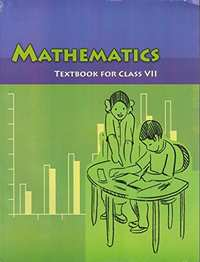## Chapter 11: Perimeter and Area

Exercise 11.1Exercise 11.2Exercise 11.3Exercise 11.4
Exercise 11.1 [Page 208]

### NCERT solutions for Class 7 Maths Chapter 11 Perimeter and AreaExercise 11.1 [Page 208]

Exercise 11.1 | Q 1.1 | Page 208

The length and the breadth of a rectangular piece of land are 500 m and 300 m respectively. Find its area

Exercise 11.1 | Q 1.2 | Page 208

The length and the breadth of a rectangular piece of land are 500 m and 300 m respectively. Find the cost of the land, if 1 m2 of the land costs Rs 10,000.

Exercise 11.1 | Q 2 | Page 208

Find the area of a square park whose perimeter is 320 m.

Exercise 11.1 | Q 3 | Page 208

Find the breadth of a rectangular plot of land, if its area is 440 m2 and the length is 22 m. Also find its perimeter.

Exercise 11.1 | Q 4 | Page 208

The perimeter of a rectangular sheet is 100 cm. If the length is 35 cm, find its breadth. Also find the area.

Exercise 11.1 | Q 5 | Page 208

The area of a square park is the same as of a rectangular park. If the side of the square park is 60 m and the length of the rectangular park is 90 m, find the breadth of the rectangular park.

Exercise 11.1 | Q 6 | Page 208

A wire is in the shape of a rectangle. Its length is 40 cm and breadth is 22 cm. If the same wire is rebent in the shape of a square, what will be the measure of each side. Also find which shape encloses more area?

Exercise 11.1 | Q 7 | Page 208

The perimeter of a rectangle is 130 cm. If the breadth of the rectangle is 30 cm, find its length. Also find the area of the rectangle.

Exercise 11.1 | Q 8 | Page 208

A door of length 2 m and breadth 1 m is fitted in a wall. The length of the wall is 4.5 m and the breadth is 3.6 m (see the given figure). Find the cost of white washing the wall, if the rate of white washing the wall is Rs 20 per m2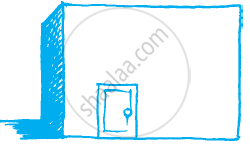Exercise 11.2 [Pages 216 - 217]

### NCERT solutions for Class 7 Maths Chapter 11 Perimeter and AreaExercise 11.2 [Pages 216 - 217]

Exercise 11.2 | Q 1.1 | Page 216

Find the area of the following parallelograms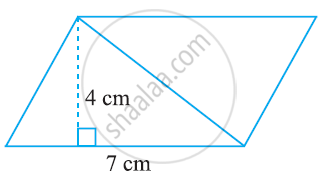Exercise 11.2 | Q 1.2 | Page 216

Find the area of the following parallelograms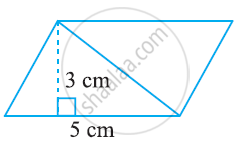Exercise 11.2 | Q 1.3 | Page 216

Find the area of the following parallelograms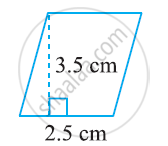Exercise 11.2 | Q 1.4 | Page 216

Find the area of the following parallelograms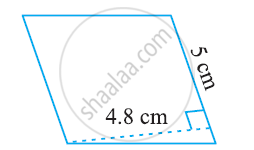Exercise 11.2 | Q 1.5 | Page 216

Find the area of the following parallelograms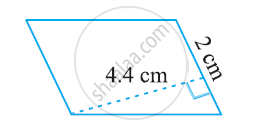Exercise 11.2 | Q 2.1 | Page 216

Find the area of the following triangles: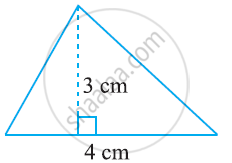Exercise 11.2 | Q 2.2 | Page 216

Find the area of the following triangles: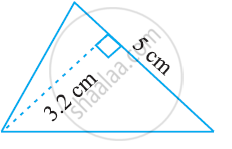Exercise 11.2 | Q 2.3 | Page 216

Find the area of the following triangles: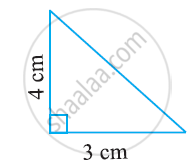Exercise 11.2 | Q 2.4 | Page 216

Find the area of the following triangles: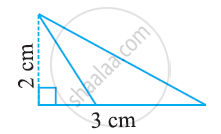Exercise 11.2 | Q 3 | Page 216

Find the missing values:

 So No Base Height Area of parallelogram a 20 cm - 246 cm2 b - 15 cm 154.5 cm2 c - 8.4 cm 48.72 cm2 d 15.6 cm - 16.38 cm2
Exercise 11.2 | Q 4 | Page 217

Find the missing values:

 Base Height Area of triangle 15 cm - 87 cm2 - 31.4 mm 1256 mm2 22 cm - 170.5 cm2
Exercise 11.2 | Q 5 | Page 217

PQRS is a parallelogram (see the given figure). QM is the height from Q to SR and QN is the height from Q to PS. If SR = 12 cm and QM = 7.6 cm. Find:

1) the area of the parallelogram PQRS

2) QN, if PS = 8 cm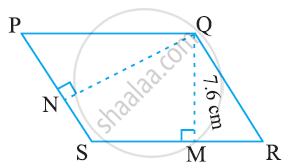Exercise 11.2 | Q 6 | Page 217

DL and BM are the heights on sides AB and AD respectively of parallelogram ABCD (see the given figure). If the area of the parallelogram is 1470 cm2, AB = 35 cm and AD = 49 cm, find the length of BM and DL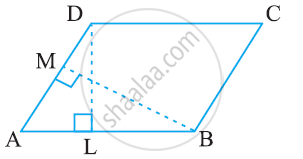Exercise 11.2 | Q 7 | Page 217

ΔABC is right angled at A (see the given figure). AD is perpendicular to BC. If AB = 5 cm, BC = 13 cm and AC = 12 cm, Find the area of ΔABC. Also find the length of AD.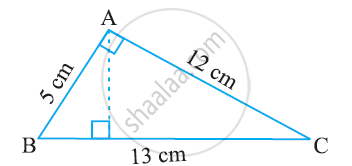Exercise 11.2 | Q 8 | Page 217

ΔABC is isosceles with AB = AC = 7.5 cm and BC = 9 cm (see the given figure). The height AD from A to BC, is 6 cm. Find the area of ΔABC. What will be the height from C to AB i.e., CE?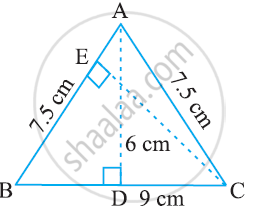Exercise 11.3 [Pages 223 - 224]

### NCERT solutions for Class 7 Maths Chapter 11 Perimeter and AreaExercise 11.3 [Pages 223 - 224]

Exercise 11.3 | Q 1.1 | Page 223

Find the circumference of the circles with the following radius (Take pi = 22/7)

14 cm

Exercise 11.3 | Q 1.2 | Page 223

Find the circumference of the circles with the following radius (Take pi = 22/7)

28 mm

Exercise 11.3 | Q 1.3 | Page 223

Find the circumference of the circles with the following radius (Take pi = 22/7)

21 cm

Exercise 11.3 | Q 2.1 | Page 223

Find the area of the following circles, given that: radius = 14 mm (take pi = 22/7)

Exercise 11.3 | Q 2.2 | Page 223

Find the area of the following circles, given that: diameter = 49 m

Exercise 11.3 | Q 2.3 | Page 223

Find the area of the following circles, given that: radius = 5 cm

Exercise 11.3 | Q 3 | Page 223

If the circumference of a circular sheet is 154m, find its radius. Also find the area of

the sheet. (take pi = 22/7)

Exercise 11.3 | Q 4 | Page 223

A gardener wants to fence a circular garden of diameter 21 m. Find the length of the rope he needs to purchase, if he makes 2 rounds of fence. Also find the costs of the rope, if it cost Rs 4 per meter (Take pi = 22/7)

Exercise 11.3 | Q 5 | Page 223

From a circular sheet of radius 4 cm, a circle of radius 3 cm is removed. Find the area of the remaining sheet. (Takeπ = 3.14)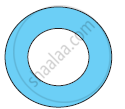Exercise 11.3 | Q 6 | Page 223

Saima wants to put a lace on the edge of a circular table cover of diameter 1.5 m. Find the length of the lace required and also find its cost if one meter of the lace costs Rs 15.( Takeπ = 3.14)

Exercise 11.3 | Q 7 | Page 223

Find the perimeter of the adjoining figure, which is a semicircle including its diameter.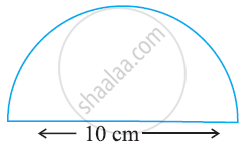Exercise 11.3 | Q 8 | Page 223

Find the cost of polishing a circular table-top of diameter 1.6 m, if the rate of polishing is Rs 15/m2. (Take π = 3.14)

Exercise 11.3 | Q 9 | Page 223

Shazli took a wire of length 44 cm and bent it into the shape of a circle. Find the radius of that circle. Also find its area. If the same wire is bent into the shape of a square, what will be the length of each of its sides? Which figure encloses more area, the circle or the square? (Take pi = 22/7)

Exercise 11.3 | Q 10 | Page 223

From a circular card sheet of radius 14 cm, two circles of radius 3.5 cm and a rectangle of length 3 cm and breadth 1 cm are removed (as shown in the following figure). Find the area of the remaining sheet (Take pi = 22/7)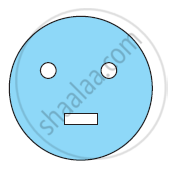Exercise 11.3 | Q 11 | Page 224

A circle of radius 2 cm is cut out from a square piece of an aluminium sheet of side 6 cm. What is the area of the left over aluminium sheet? (Take π = 3.14)

Exercise 11.3 | Q 12 | Page 224

The circumference of a circle is 31.4 cm. Find the radius and the area of the circle? (Take π = 3.14)

Exercise 11.3 | Q 13 | Page 224

A circular flower bed is surrounded by a path 4 m wide. The diameter of the flower bed is 66 m. What is the area of this path? (π = 3.14)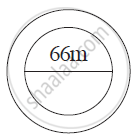Exercise 11.3 | Q 14 | Page 224

A circular flower garden has an area of 314 m2. A sprinkler at the centre of the garden can cover an area that has a radius of 12 m. Will the sprinkler water the entire garden? (Take π = 3.14)

Exercise 11.3 | Q 15 | Page 224

Find the circumference of the inner and the outer circles, shown in the adjoining figure? (Take π = 3.14)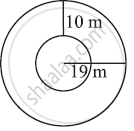Exercise 11.3 | Q 16 | Page 224

How many times a wheel of radius 28 cm must rotate to go 352 m? (Take pi = 22/7)

Exercise 11.3 | Q 17 | Page 224

The minute hand of a circular clock is 15 cm long. How far does the tip of the minute hand move in 1 hour. (Take π = 3.14)

Exercise 11.4 [Pages 226 - 227]

### NCERT solutions for Class 7 Maths Chapter 11 Perimeter and AreaExercise 11.4 [Pages 226 - 227]

Exercise 11.4 | Q 1 | Page 226

A garden is 90 m long and 75 m broad. A path 5 m wide is to be built outside and around it. Find the area of the path. Also find the area of the garden in hectare

Exercise 11.4 | Q 2 | Page 226

A 3 m wide path runs outside and around a rectangular park of length 125 m and breadth 65 m. Find the area of the path.

Exercise 11.4 | Q 3 | Page 226

A picture is painted on a cardboard 8 cm long and 5 cm wide such that there is a margin of 1.5 cm along each of its sides. Find the total area of the margin.

Exercise 11.4 | Q 4 | Page 226

A verandah of width 2.25 m is constructed all along outside a room which is 5.5 m long and 4 m wide. Find:

1) the area of the verandah

2) the cost of cementing the floor of the verandah at the rate of Rs 200 per m2.

Exercise 11.4 | Q 5 | Page 226

A path 1 m wide is built along the border and inside a square garden of side 30 m. Find:

1) the area of the path

2) the cost of planting grass in the remaining portion of the garden at the rate of Rs 40 per m2

Exercise 11.4 | Q 6 | Page 227

Two cross roads, each of width 10 m, cut at right angles through the centre of a rectangular park of length 700 m and breadth 300 m and parallel to its sides. Find the area of the roads. Also find the area of the park excluding cross roads. Give the answer in hectares.

Exercise 11.4 | Q 7 | Page 227

Through a rectangular field of length 90 m and breadth 60 m, two roads are constructed which are parallel to the sides and cut each other at right angles through the centre of the fields. If the width of each road is 3 m, find

1)the area covered by the roads.

2) the cost of constructing the roads at the rate of Rs 110 per m2.

Exercise 11.4 | Q 8 | Page 227

Pragya wrapped a cord around a circular pipe of radius 4 cm (adjoining figure) and cut off the length required of the cord. Then she wrapped it around a square box of side 4 cm (also shown). Did she have any cord left? (π= 3.14)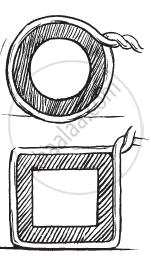Exercise 11.4 | Q 9 | Page 227

The adjoining figure represents a rectangular lawn with a circular flower bed in the
middle. Find:

1) the area of the whole land

2) the area of the flower bed

3) the area of the lawn excluding the area of the flower bed

4)  the circumference of the flower bed.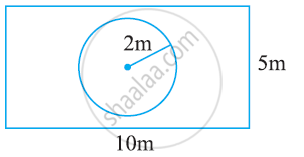Exercise 11.4 | Q 10.1 | Page 227

In the following figure, find the area of the shaded portions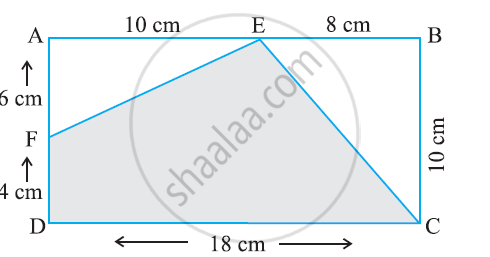Exercise 11.4 | Q 10.2 | Page 227

In the following figure, find the area of the shaded portions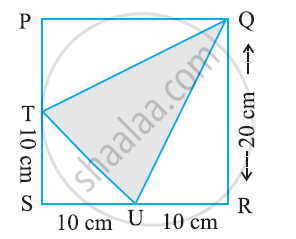Exercise 11.4 | Q 11 | Page 227

Find the area of the quadrilateral ABCD.

Here, AC = 22 cm, BM = 3 cm,

DN = 3 cm, and

BM⊥AC, DN⊥AC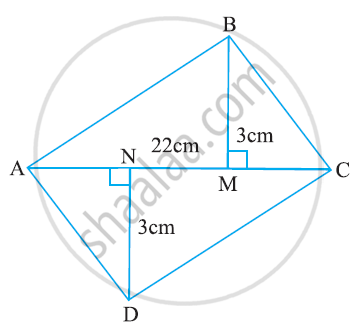## Chapter 11: Perimeter and Area

Exercise 11.1Exercise 11.2Exercise 11.3Exercise 11.4## NCERT solutions for Class 7 Maths chapter 11 - Perimeter and Area

NCERT solutions for Class 7 Maths chapter 11 (Perimeter and Area) include all questions with solution and detail explanation. This will clear students doubts about any question and improve application skills while preparing for board exams. The detailed, step-by-step solutions will help you understand the concepts better and clear your confusions, if any. Shaalaa.com has the CBSE Class 7 Maths solutions in a manner that help students grasp basic concepts better and faster.

Further, we at Shaalaa.com provide such solutions so that students can prepare for written exams. NCERT textbook solutions can be a core help for self-study and acts as a perfect self-help guidance for students.

Concepts covered in Class 7 Maths chapter 11 Perimeter and Area are Area of Square, Triangles as Parts of Rectangles and Square, Generalising for Other Congruent Parts of Rectangles, Mensuration, Concept of Perimeter, Perimeter of Polygon, Area of Rectangle, Circumference of a Circle, Perimeter of a Rectangle, Perimeter of Squares, Perimeter of Triangles, Problems based on Perimeter and Area, Concept of Area, Area of a Triangle, Area of a Parallelogram, Area of Circle, Conversion of Units, Problems based on Perimeter and Area.

Using NCERT Class 7 solutions Perimeter and Area exercise by students are an easy way to prepare for the exams, as they involve solutions arranged chapter-wise also page wise. The questions involved in NCERT Solutions are important questions that can be asked in the final exam. Maximum students of CBSE Class 7 prefer NCERT Textbook Solutions to score more in exam.

Get the free view of chapter 11 Perimeter and Area Class 7 extra questions for Class 7 Maths and can use Shaalaa.com to keep it handy for your exam preparation